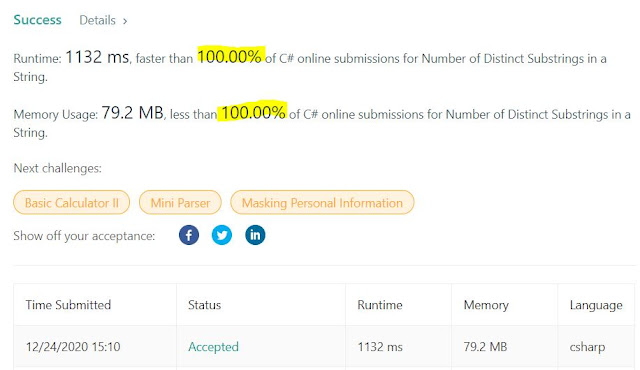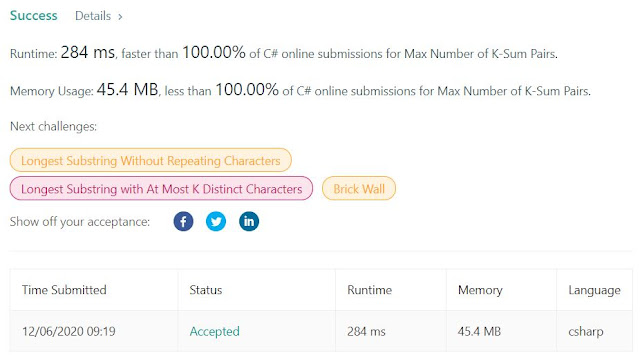## Posts

Showing posts from December, 2020

### Trie Variation for a Hard Leetcode ProblemHere is the problem:  Number of Distinct Substrings in a String - LeetCode 1698. Number of Distinct Substrings in a String Hard 4 0 Add to List Share Given a string  s , return  the number of  distinct  substrings of   s . A  substring  of a string is obtained by deleting any number of characters (possibly zero) from the front of the string and any number (possibly zero) from the back of the string.   Example 1: Input: s = "aabbaba" Output: 21 Explanation: The set of distinct strings is ["a","b","aa","bb","ab","ba","aab","abb","bba","aba","aabb","abba","bbab","baba","aabba","abbab","bbaba","aabbab","abbaba","aabbaba"] Example 2: Input: s = "abcdefg" Output: 28   Constraints: 1 <= s.length <= 500 s  consists of lowercase English letters. Accepted 118 Submissio

### Two Pointers for a Linear Solution IVI've written before about  two-pointers techniques  before. In this particular case (Medium LC), you'll need to use some sort of hash or memo to keep track of all the unique values, but the two-pointers solution remains standard. Here it is:  Maximum Erasure Value - LeetCode You are given an array of positive integers  nums  and want to erase a subarray containing  unique elements . The  score  you get by erasing the subarray is equal to the  sum  of its elements. Return  the  maximum score  you can get by erasing  exactly one  subarray. An array  b  is called to be a  subarray  of  a  if it forms a contiguous subsequence of  a , that is, if it is equal to  a[l],a[l+1],...,a[r]  for some  (l,r) .   Example 1: Input: nums = [4,2,4,5,6] Output: 17 Explanation: The optimal subarray here is [2,4,5,6]. Example 2: Input: nums = [5,2,1,2,5,2,1,2,5] Output: 8 Explanation: The optimal subarray here is [5,2,1] or [1,2,5].   Constraints: 1 <= nums.length <= 10 5 1 <= nums[i

### Advent Of Code Day 10: Backtracking and Dynamic ProgrammingHowdy Everyone,   Catching up on the Advent of Code, I got to day 10 today, here it is:  Day 10 - Advent of Code 2020 .  Description is a little long and tedious but in the end you get the gist of it. Part 1 is looking for a simple path from 0 to max. I looked at the input size and it did not seem that large, so I thought backtracking would do the trick. Indeed a simple straightforward DFS backtracking from 0 to max works. Part 2 asks for the count of ALL paths. That's a little tricky, especially after reading this part of the description: You glance back down at your bag and try to remember why you brought so many adapters; there must be  more than a trillion  valid ways to arrange them! Surely, there must be  an efficient way  to count the arrangements. That should give you the hint that the "adventers" are looking for a Dynamic Programming (DP) solution. Luckily, this DP is super simple, and the way that I like to implement DP is by construction from 0..max. Basically

### Advent of Code - Day 8: Handheld Halting

Tricky one, resembling more like a medium LC problem. Here it is:  Day 8 - Advent of Code 2020 Especially the second part of the problem: --- Part Two --- After some careful analysis, you believe that  exactly one instruction is corrupted . Somewhere in the program,  either  a  jmp  is supposed to be a  nop ,  or  a  nop  is supposed to be a  jmp . (No  acc  instructions were harmed in the corruption of this boot code.) The program is supposed to terminate by  attempting to execute an instruction immediately after the last instruction in the file . By changing exactly one  jmp  or  nop , you can repair the boot code and make it terminate correctly. For example, consider the same program from above: nop +0 acc +1 jmp +4 acc +3 jmp -3 acc -99 acc +1 jmp -4 acc +6 If you change the first instruction from  nop +0  to  jmp +0 , it would create a single-instruction infinite loop, never leaving that instruction. If you change almost any of the  jmp  instructions, the program will still eventu

### Two Pointers for a Linear Solution III (actually, NLogN)I've written before about two-pointers techniques  before. Usually this technique works well with sorted array: have the first pointer in the very left, second pointer in the very right, and do the processing until the pointers cross each other. If the array is already sorted, the solution is linear, otherwise you'll end up with an NLogN. This problem exemplifies the technique:  Max Number of K-Sum Pairs - LeetCode 1679. Max Number of K-Sum Pairs Medium 46 4 Add to List Share You are given an integer array  nums  and an integer  k . In one operation, you can pick two numbers from the array whose sum equals  k  and remove them from the array. Return  the maximum number of operations you can perform on the array .   Example 1: Input: nums = [1,2,3,4], k = 5 Output: 2 Explanation: Starting with nums = [1,2,3,4]: - Remove numbers 1 and 4, then nums = [2,3] - Remove numbers 2 and 3, then nums = [] There are no more pairs that sum up to 5, hence a total of 2 operations. Example 2:

### Advent of Code 5: Binary BoardingThis was an interesting one, but still comparable to an "easy" Leetcode problem (I'm sure this will get tougher as the days go by):  Day 5 - Advent of Code 2020 The analysis of each seat is a variation of a Binary Search . What I did is one function that given a string returns the number associated with it. That way you can call it for the 0-6 and 7-9 parts of the string. After that, the first part is easy, just getting the max. The second part is also relatively easy: sort the entries, and then go one by one from the very beginning looking for the first gap. Once found, that's your solution. Code is down below, cheers, ACC. static void Main(string[] args) { FileInfo fi = new FileInfo(args); StreamReader sr = fi.OpenText(); List list = new List (); int max = 0; while (!sr.EndOfStream) { string str = sr.ReadLine().Trim(); if (!String.IsNullOrEmpty(str)) { int val = SeatNumber(str.Substring(0, 7)) * 8 + Sea

### Advent of Code - Day 3

Super cool the Advent of Code - something fun to do in the last 30+ days of the year. Check it out. Two challenges every night for a little over a month:  Day 3 - Advent of Code 2020 For day #3, best is to push the input to a list of strings, and then create a function that takes that list, the col and the row, looking for the trees. Some modular math in the middle to account for the infinite cols. First submission failed due to the fact that the product will be larger than Int.Max. Switch to long, and it works. Fun idea. Code is below, no spoillers (won't give away the result). Cheers, ACC. using System; using System.IO; using System.Collections; using System.Collections.Generic; using System.Text; namespace AdventOfCode { class Program { static void Main(string[] args) { FileInfo fi = new FileInfo(args); StreamReader sr = fi.OpenText(); List input = new List (); while (!sr.EndOfStream) {